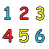Can you use the digits 1, 2, 3, 4, 5 and 6, each only once, to make a number which is divisible by 6
… so that when the final or last digit is removed it becomes a 5-digit number divisible by 5,
and when the last digit is removed from that number it becomes a 4-digit number divisible by 4,
and when the last digit is removed from that number it becomes a 3-digit number divisible by 3,
and when again the last digit is removed from that number it becomes a 2-digit number divisible by 2, then finally a 1-digit number divisible by 1?
You might start by trying the same puzzle with 3 digits, then 4 digits, then 5 digits before solving the bigger puzzle with 6 digits. After that perhaps generalise the puzzle and think about more digits.

Tagged with:

### 2 Responses to Curious Number

1.Stombles Magadlela says:

The will be 213456.this number is divisible by by 6

2.Cynthia Fries says:

Well done Nelly. Did your class find the second solution?
Stombles, 213456 is divisible by 6 and 21345 is divisible by 5, but 2134 is not divisible by 4. If you click on the link ‘notes for teachers’ written in blue at the bottom of the problem, you will see the notes that Toni has written for you all to use. We would like you to use these problems in your classes then come back to this page and tell us all about it. Which class did you think this was suitable for? How did you introduce it? Was it a successful lesson? Are there any hints or advice you would like to pass on to other teachers?